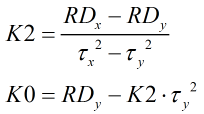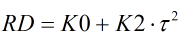# C258 – Gas Relative Density Calculation – Solartron

## Description

This calculates transducer constants K0 and K2 with the option of performing a cross check on the density calculation.

Solartron NT3096 Specific Gravity Transducer Technical Manual Part No 30960015 Issue 5 August 1984

Kelton calculation reference C258

FLOCALC calculation reference F061

KIMS calculation reference K204

## Options

### Solve For

• Transducer constants
• Relative density

If Relative density is selected the following option box will appear

### Transducer Constants

• User Entered
• Calculated

Select options depending on what needs to be calculated.

## Calculation

### Transducer Constants

The transducer constants are calculated by:Where RDx = Relative density of calibration gas x RDy = Relative density of calibration gas y τx = Periodic time associated with calibration gas x τy = Periodic timeassociated with calibration gas y

### Relative Density

The relative density is calculated by:K0 & K2 = Transducer constants τ = Periodic time associated with the measured gas

Back